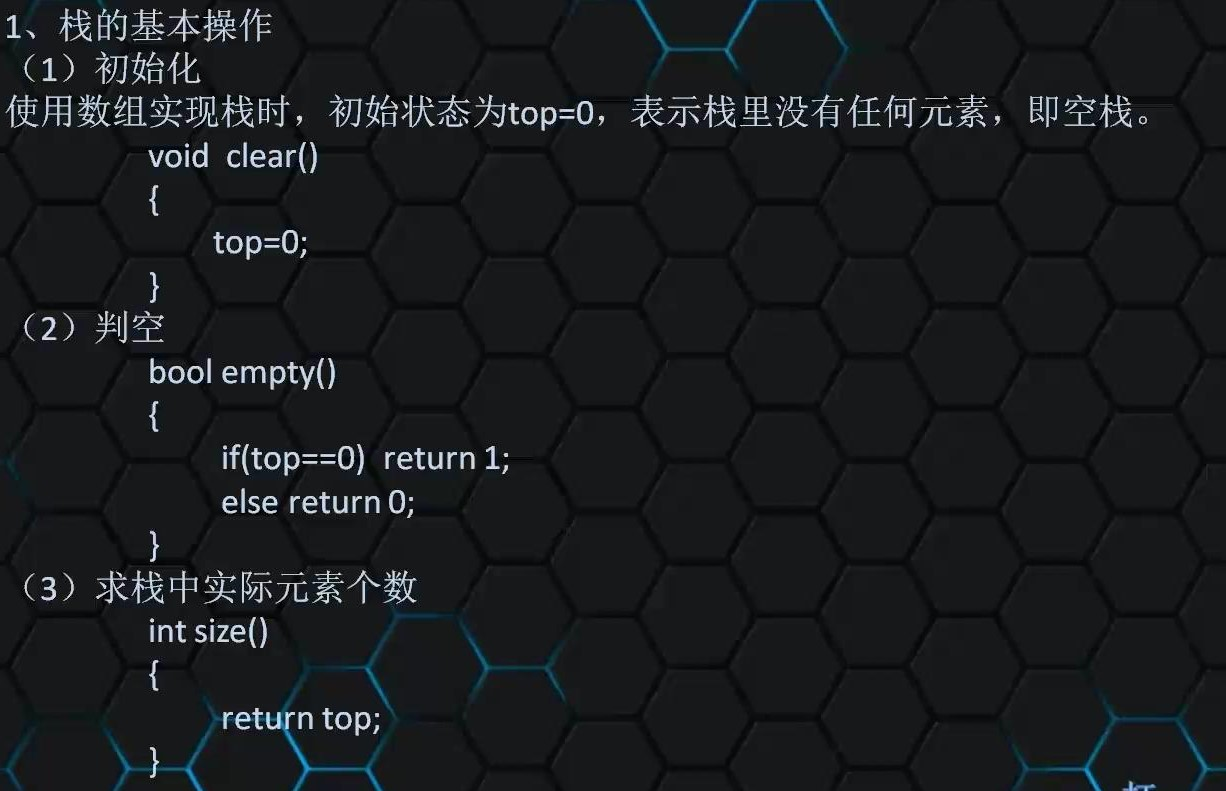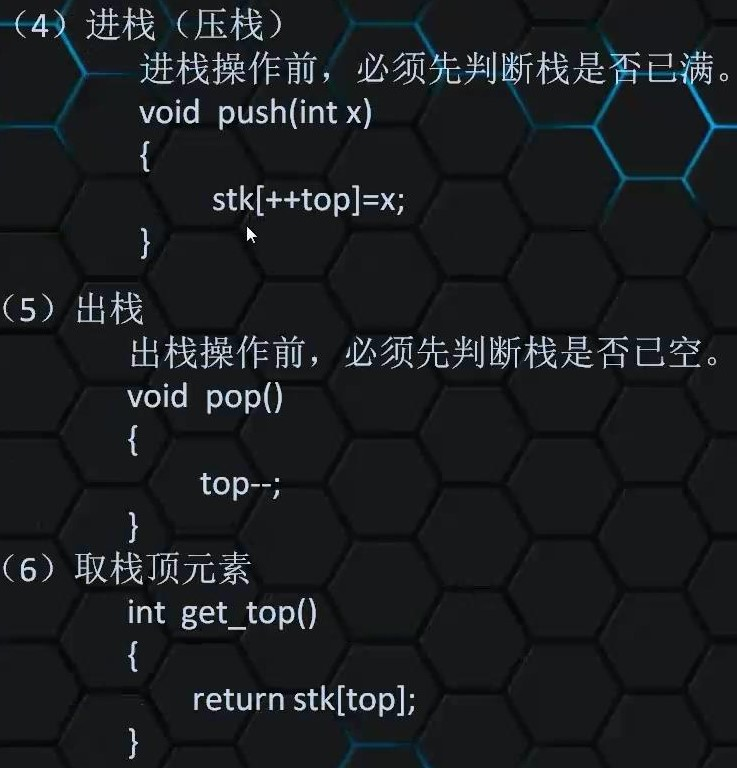# 数据结构基础

## 二.线性结构### 代码展示：

``````#include<bits/stdc++.h>
using namespace std;
long long n,a,m,number;
void Insert(int x,int y)
{
for(int i=n;i>=x;i--)
a[i+1]=a[i];
a[x]=y;
}
void Delete(int x)
{
for(int i=1;i<=n;i++)
a[i-1]=a[i];
}
int main()
{
cin>>n;
for(int i=1;i<=n;i++)
cin>>a[i];
cin>>m;
for(int i=1;i<=m;i++)
{
int x,y;
cin>>number;
if(number==1)
{
cin>>x;
cout<<a[x];
}
else if(number==2)
{
cin>>x>>y;
Insert(x,y);
n++;
}
else if(number==3)
{
cin>>x;
Delete(x);
}
}
return 0;
}``````